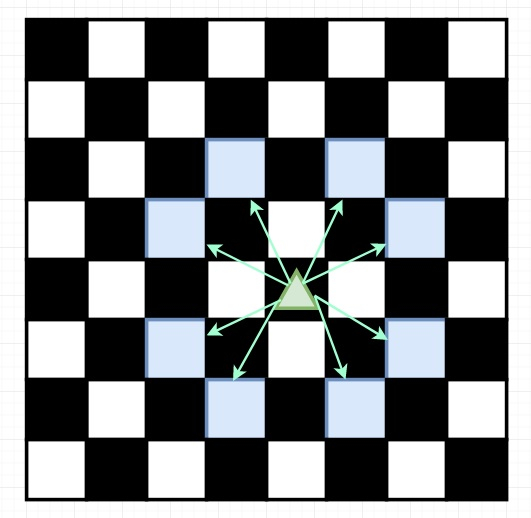# Knight Probability in Chessboard in C++

Suppose we have one NxN chessboard, a knight starts at the r-th row and c-th column and attempts to make exactly K moves. Here the rows and columns are 0 indexed, so the top-left square is (0, 0), and the bottom-right square is (N-1, N-1).

A knight can move in 8 different cells from a cell, that can be shown in this diagram −Each time the knight is to move, it chooses one of eight possible moves randomly. The knight continues moving until it has made exactly K moves or has moved off the chessboard. We have to find the probability that the knight remains on the board after it has stopped moving.

So if the input is like 3, 2, 0, 0, then the output will be 0.0625. This is because there are two moves (to (1,2), (2,1)) that will keep the knight on the board. Now from each of those positions, there are also two moves that will keep the knight on the board. So here the total probability the knight stays on the board is 0.0625.

To solve this, we will follow these steps −

• Define one direction array dir, this is like [[-2,-1], [-2, 1],[2,-1], [2, 1], [1,2], [1,-2], [-1,2], [-1,-2]]
• Define recursive method solve(), this will take x, y, n, k, and 3d array dp
• if x >= n or y >= n or x < 0 or y < 0, then return 0
• if k is 0, then return 1
• if dp[k,x,y] is not -1, then return dp[k,x,y]
• dp[k, x, y] := 0
• for i in range 0 to 7
• dp[k,x,y] := solve(x+dir[i,0], y + dir[i, 1], n, k – 1, dp)
• return dp[k,x,y]
• From the main method, do the following
• make a 3d array of size (k + 1) x N x N. Fill this with – 1
• return solve(r, c, N, k, dp) / (8^K)

Let us see the following implementation to get better understanding −

## Example

Live Demo

#include <bits/stdc++.h>
using namespace std;
int dir = {{-2, -1}, {-2, 1}, {2, -1}, {2, 1}, {1, 2}, {1, -2}, {-1, 2}, {-1, -2}};
class Solution {
public:
double solve(int x, int y, int n, int k, vector < vector < vector < double > > >& dp){
if(x >= n || y >= n || x < 0 || y < 0 ) return 0.0;
if(k == 0) return 1.0;
if(dp[k][x][y] != -1) return dp[k][x][y];
dp[k][x][y] = 0;
for(int i = 0; i < 8; i++){
dp[k][x][y] += solve(x + dir[i], y + dir[i], n, k - 1, dp);
}
return dp[k][x][y];
}
double knightProbability(int N, int K, int r, int c) {
vector < vector < vector < double > > > dp (K + 1, vector < vector < double > >(N, vector < double >(N, -1))) ;
return solve(r, c, N, K, dp) / pow(8, K);
}
};
main(){
Solution ob;
cout << (ob.knightProbability(3, 2, 0, 0));
}

## Input

3
2
0
0

## Output

0.0625